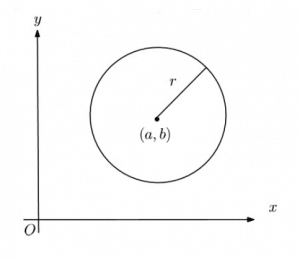# Coordinate Geometry in A-Level Maths

## Coordinate GeometryWhen we study shapes in a coordinate plane, we call it Coordinate Geometry.  The majority of what we study in Coordinate Geometry, we will do in the Cartesian plane. This is when we have an $x$ and $y$ axis and we describe points by their $(x,y)$ coordinates. At more advanced levels, it is possible to describe shapes with polar coordinates (see more on polar coordinates).

In many cases, a coordinate geometry question will be presented with an accompanying diagram. However, if it is not, it is always a good idea to make your own diagram. Even if there is not enough information to create an accurate diagram, make the best sketch that you can.

You should have actually studied some coordinate geometry before. At GCSE, we learn the equation of a straight line (as well as other curves such as quadratics). Primarily, equations of Straight Lines and equations of Circles and the interesting problems that come with them are covered in the first year of A-Level Maths. Furthermore, we introduce Parametric Equations in the second year.